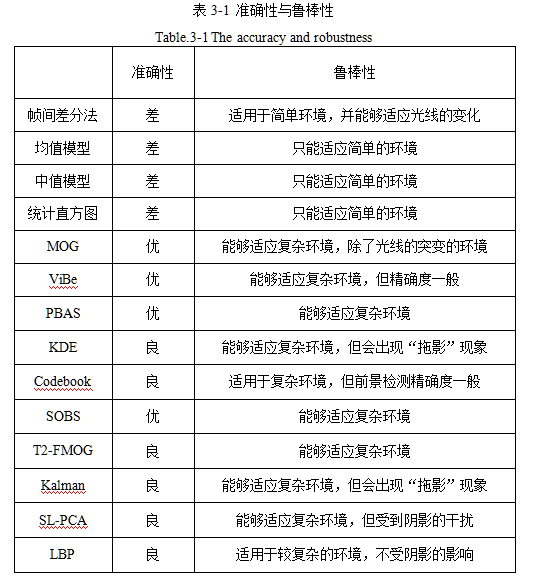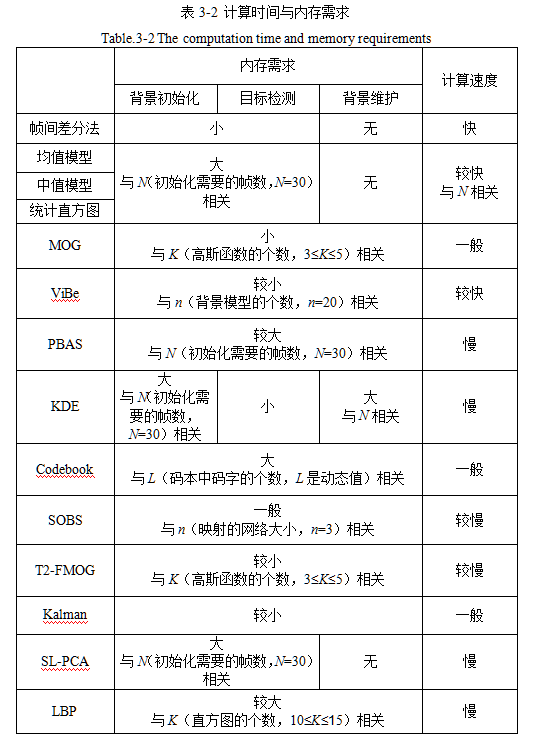# 基于Python的OpenCV图像处理18

## Jun 30,2017   14865 words   54 min

#### 一、灰度随时间的变化

# coding=utf-8
import cv2
from matplotlib import pyplot as plt

path = 'E:\\vtest.avi'

cap = cv2.VideoCapture(path)

width = int(cap.get(3))
height = int(cap.get(4))

r_list = []
g_list = []
b_list = []
gray_list = []

while 1:
if frame is None:
cv2.waitKey(0)
break
else:
b, g, r = frame[int(width / 2), int(height / 2), :].ravel()
b_list.append(b)
g_list.append(g)
r_list.append(r)
gray_list.append((r * 30 + g * 59 + b * 11 + 50) / 100)
print 'R:', r_list[-1], 'G:', g_list[-1], 'B:', b_list[-1], 'Gray:', gray_list[-1]

cv2.imshow('frame', frame)

k = cv2.waitKey(50) & 0xff
if k == 27:
break

plt.plot(r_list, color='red', label='Red')
plt.plot(g_list, color='green', label='Green')
plt.plot(b_list, color='blue', label='Blue')
plt.plot(gray_list, color='gray', label='Gray')
plt.legend(loc='upper right')
plt.show()

cap.release()
cv2.destroyAllWindows()


#### 二、OpenCV图像数据类型与代数运算

##### 1.加法

uint8范围为0-255。采用OpenCV内置加法进行相加时，如果超限，则以255代替。如下图所示。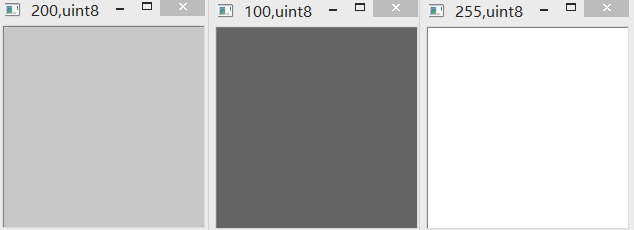###### (2)“+” & uint8

uint8范围为0-255。采用普通加法相加，如果超限，则减去256(即该范围包含的数字个数)。如下图所示。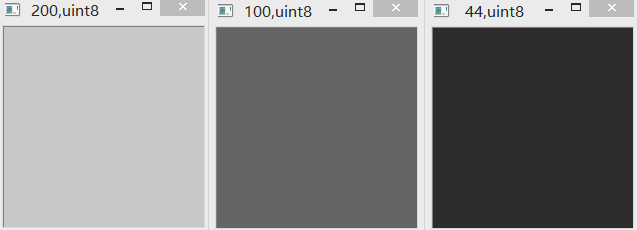int8范围为-128到127。采用OpenCV加法相加，如果超限，则以最大值127代替。如下图所示。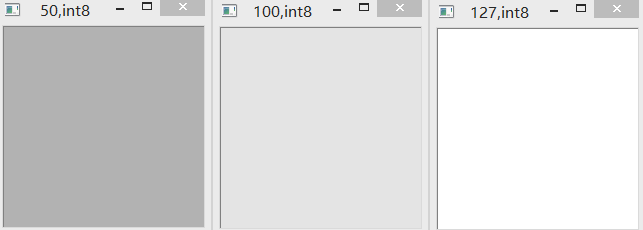###### (4)“+” & int8

int8范围为-128到127。采用普通加法相加，如果超限，则减去256(即该范围包含的数字个数)。如下图所示。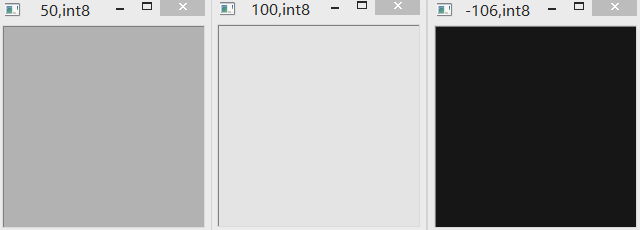###### (5)cv2.subtract & uint8

uint8范围为0-255。采用OpenCV减法相减，如果超限，则以最小值0代替。如下图所示。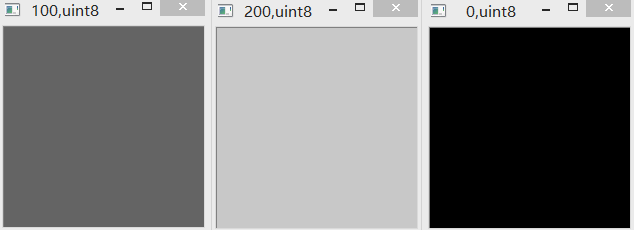###### (6)“-” & uint8

uint8范围为0-255。采用普通减法相减，如果超限，则以加上256(即该范围包含的数字个数)。如下图所示。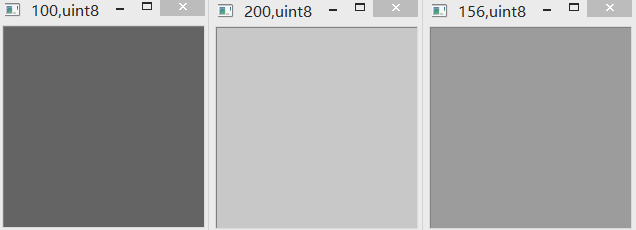###### (7)cv2.subtract & int8

int8范围为-128到127。采用OpenCV减法相减，如果超限，则以最小值-128代替。如下图所示。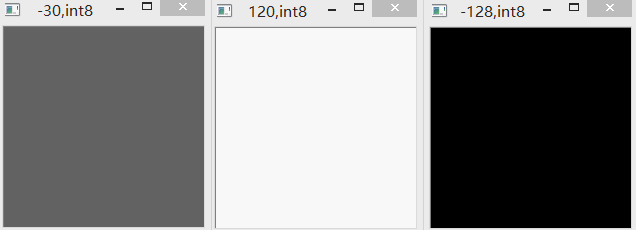###### (8)“-” & int8

int8范围为-128到127。采用普通减法相减，如果超限，则以加上256(即该范围包含的数字个数)。如下图所示。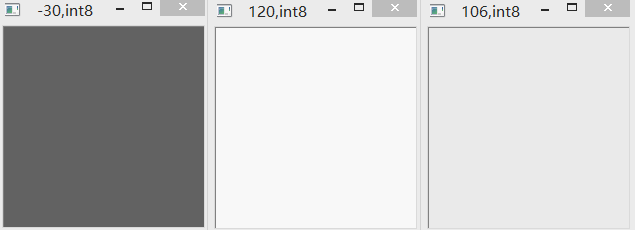import cv2
import numpy as np

img1 = np.zeros((200, 200, 3), np.int8)
img1[...] = -30

img2 = np.zeros((200, 200, 3), np.int8)
img2[...] = 120

# img3 = img1 + img2
# img3 = cv2.subtract(img1, img2)
img3 = img1 - img2

cv2.imshow(img1[0, 0, 0].__str__() + ',' + img1.dtype.__str__(), img1)
cv2.imshow(img2[0, 0, 0].__str__() + ',' + img1.dtype.__str__(), img2)
cv2.imshow(img3[0, 0, 0].__str__() + ',' + img1.dtype.__str__(), img3)
cv2.waitKey(0)

###### (9)相减获得绝对值

import cv2
import numpy as np

img1 = np.zeros((200, 200, 3), np.uint8)
img1[...] = 90

img2 = np.zeros((200, 200, 3), np.uint8)
img2[...] = 200

img3 = np.uint8(np.abs(np.int32(img1) - np.int32(img2)))

cv2.imshow(img1[0, 0, 0].__str__() + ',' + img1.dtype.__str__(), img1)
cv2.imshow(img2[0, 0, 0].__str__() + ',' + img1.dtype.__str__(), img2)
cv2.imshow(img3[0, 0, 0].__str__() + ',' + img1.dtype.__str__(), img3)
cv2.waitKey(0)


#### 三、背景减除

##### 0.最初的想法

# coding=utf-8
import cv2
import numpy as np

path = 'E:\\vtest.avi'

cap = cv2.VideoCapture(path)

width = int(cap.get(3))
height = int(cap.get(4))

initial = np.zeros((height, width, 3), np.uint8)

while 1:
if frame is None:
cv2.waitKey(0)
break
else:
# 两帧相减
sub = cv2.subtract(initial, frame)
# 将当前帧赋给循环变量作为上一帧
initial = frame

cv2.imshow('frame', sub)
k = cv2.waitKey(30) & 0xff
if k == 27:
break

cap.release()
cv2.destroyAllWindows()


# coding=utf-8
import cv2
import numpy as np

path = 'E:\\vtest.avi'

cap = cv2.VideoCapture(path)

width = int(cap.get(3))
height = int(cap.get(4))

initial = np.zeros((height, width, 3), np.uint8)

while 1:
if frame is None:
cv2.waitKey(0)
break
else:
# 两帧相减
sub = np.uint8(np.abs(np.int32(initial) - np.int32(frame)))
# 将当前帧赋给循环变量作为上一帧
initial = frame

cv2.imshow('F', sub)
k = cv2.waitKey(30) & 0xff
if k == 27:
break

cap.release()
cv2.destroyAllWindows()


##### 2.OpenCV中的方法

###### (2)BackgroundSubtractorMOG2

# coding=utf-8
import cv2

path = 'E:\\vtest.avi'

cap = cv2.VideoCapture(path)

# 新建一个MOG背景减除对象
fgbg = cv2.createBackgroundSubtractorMOG2()

# 新建一个卷积核用于开运算
kernel = cv2.getStructuringElement(cv2.MORPH_RECT, (3, 3))

while 1:
if frame is None:
cv2.waitKey(0)
break
else:
# 应用MOG到每一帧，返回结果是动目标的掩膜
# 利用开运算进行噪声去除

k = cv2.waitKey(30) & 0xff
if k == 27:
break

cap.release()
cv2.destroyAllWindows()


##### 3.较完善的程序

# coding=utf-8
import cv2

path = ''
path = raw_input("Path of input video:\n")

output = ''
output = raw_input("Path of output video('None' means don't save):\n")

noiseFilter = 1
noiseFilter = input("Whether use morphological opening to remove noise.0 means no,1 means yes:\n")

showVideo = 1
showVideo = input("Whether show video during processing.0 means no,1 means yes:\n")

outputType = 1
outputType = input("Select type of output video.\n0:mask video\n1:subtraction video\n")

method = 0
method = input("Select method for background subtraction.\n0:MOG2 method\n1:KNN method\n")

subtractMode = 1
subtractMode = input(

cap = cv2.VideoCapture(path)

width = int(cap.get(3))
height = int(cap.get(4))
total = int(cap.get(7))
count = 0

if method is 0:
if subtractMode is 1 or 2:
fgbg = cv2.createBackgroundSubtractorMOG2()
elif subtractMode is 3:
elif method is 1:
if subtractMode is 1 or 2:
fgbg = cv2.createBackgroundSubtractorKNN()
elif subtractMode is 3:

kernel = cv2.getStructuringElement(cv2.MORPH_RECT, (3, 3))

fps = 10
waitTime = 1
if cap.get(5) != 0:
waitTime = int(1000.0 / cap.get(5))
fps = cap.get(5)

if output is not "None":
fourcc = cv2.VideoWriter_fourcc(*'XVID')
out = cv2.VideoWriter(output, fourcc, fps, (width, height))

while 1:
if frame is None:
cv2.waitKey(0)
break
else:

if subtractMode is 2:

if noiseFilter is 1:

if outputType is 1:
if showVideo is 1:
cv2.imshow('frame', dst)
if output is not "None":
out.write(dst)
elif outputType is 0:
if showVideo is 1:
if output is not "None":

count += 1
print (count * 1.0 / total) * 100, "% finished."
k = cv2.waitKey(30) & 0xff
if k == 27:
break

cap.release()
out.release()
cv2.destroyAllWindows()


• 待处理视频路径，如”E:\vtest.avi”
• 输出视频路径，如”C:\output.avi”，”None”代表不输出视频
• 是否使用形态学开运算去除噪声，0-不使用，1-使用
• 是否在处理时显示视频，0-不显示，1-显示
• 输出视频类型，0-掩膜，1-掩膜后的视频内容
• 背景减除算法，0-MOG2,1-KNN
• 减除模式，1-检测并显示阴影，2-检测并去除阴影，3-不检测阴影

#### 四、背景减除方法综述

##### 1.简单背景建模方法

$B_{t}(i,j)=\frac{1}{N}\sum_{t=0}^{N}I_{t}(i,j)$ $X_{t}(i,j)=\left\{\begin{matrix} 1,|I_{t}(i,j)-B_{t}(i,j)|>T\\ 0,|I_{t}(i,j)-B_{t}(i,j)|\leq T \end{matrix}\right.$

# coding=utf-8
import cv2
import numpy as np

# 利用简单背景建模法提取背景(均值模型)

cap = cv2.VideoCapture("E:\\vtest.avi")

# 获取视频宽高以及总帧数
width = int(cap.get(3))
height = int(cap.get(4))
total = int(cap.get(7))

# 注意数据类型对应的范围
# 由于各帧相加会得到很大的数，因此先使用int32类型
average = np.zeros((height, width, 3), np.int32)

while cap.isOpened():
if frame is None:
break
else:
cv2.imshow("video", frame)
# 对每一帧进行累加
average += frame
k = cv2.waitKey(30) & 0xFF
if k == 27:
break

# 将累加结果除以总帧数
average = np.uint8(average / total)
cv2.imshow("background", average)
cv2.waitKey(0)
cap.release()


# coding=utf-8
import cv2
import numpy as np

# 利用简单背景建模法提取前景(均值模型)

cap = cv2.VideoCapture("E:\\vtest.avi")

# 获取视频宽高以及总帧数
width = int(cap.get(3))
height = int(cap.get(4))
total = int(cap.get(7))

count = 0

# 注意数据类型对应的范围
# 由于各帧相加会得到很大的数，因此先使用int32类型
average = np.zeros((height, width, 3), np.int32)

while cap.isOpened():
if frame is None:
break
else:
count += 1
print (count * 1.0 / total) * 100, '%'
# 对每一帧进行累加
average += frame
k = cv2.waitKey(30) & 0xFF
if k == 27:
break

# 将累加结果除以总帧数
average = average / total
cap.release()

# 重新打开视频进行前景提取
cap2 = cv2.VideoCapture("E:\\vtest.avi")
while cap2.isOpened():
if frame is None:
cv2.waitKey(0)
break
else:
# 每一帧与背景相减
# 注意这里不要使用OpenCV的减法，因为数据类型不同
# 首先将两帧相减，得到int32型的数据，然后再取绝对值，再化为uint8
# 对前景掩膜灰度化然后再二值化得到最终掩膜
# 与每一帧进行与操作，获取内容
cv2.imshow("thresh=40", foreground)

k = cv2.waitKey(30) & 0xFF
if k == 27:
break
cap2.release()


##### 2.基于统计的背景建模方法

###### a.模型初始化

$P(X_{t})=\sum_{k=1}^{K}\omega _{k}\cdot \eta (X_{t},\mu _{k},\sum_{ }{ }_{k})$

$\eta (X_{t},\mu _{k},\sum_{ }{ }_{k})=\frac{1}{|\sum_{ }{ }_{k}|^{\frac{1}{2}}}e^{-\frac{1}{2}(x-\mu _{k})^{T}\sum_{ }{ }_{k}^{-1}(x-\mu _{k})}\\ \sum_{ }{ }_{k}=\sigma _{k}^{2}I$

###### b.目标检测

$B=arg(min(\sum_{j=1}^{b}\omega _{j}>T))$

$|X_{N}-\mu _{k}|<2.5\sigma _{k}$
###### c.参数更新

$\omega _{k}^{'}=(1-\alpha)\omega_{k}+\alpha\rho \\ \mu_{k}^{'}=(1-\rho)\mu_{k}+\rho X_{t}\\ \sigma_{k}^{2^{'}}=(1-\rho)\sigma_{k}^{2}+\rho(X_{t}-\mu_{k})^{T}(X_{t}-\mu_{k})$

##### 3.基于聚类的背景建模法

CodeBook算法是一种经典的基于聚类的背景建模方法。该算法从一长串视频序列中建立背景模型，并为每个像素建立一个包含一个或多个码字的CodeBook。 码字中存放着每个像素的色彩失真量和亮度区间。通过色彩失真量和亮度区间来区分背景和前景。

##### 4.基于自组织神经网络的背景建模法

SOBS是一种基于自组织神经网络的背景减除算法。该方法将视频帧映射成一幅神经网络背景模型图，并考虑了相邻像素间的空间相关性。 此外，该方法选择HSV颜色空间进行建模，由于它能很好地分离亮度和色调信息，这使得运动检测结果不受光线影响。

##### 7.各类方法比较